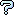All about flooble | fun stuff | Get a free chatterbox | Free JavaScript | Avatarsperplexus dot infoTipping Tube (Posted on 2005-06-23)A man places a circular tube upright on a table. He then places a solid ball into the tube followed by a smaller solid ball. The tube stays upright. He removes the balls and places them again with the smaller ball being placed in first. The tube tips over. In both cases the man holds onto the tube until the balls come to rest and then lets go. The radii of the balls are 2.6 and 3.4 centimeters. The length of the tube is 18.0 centimeters and its thickness (external radius minus internal radius) is 0.1 centimeter. The balls and tube are made of the same material - so their weights are proportional to their volumes. Assume the points of contact between the balls, table, and tube are frictionless. What are the minimum and maximum values for the internal radius of the tube?

 Submitted by Bractals Rating: 3.7500 (4 votes) Solution: (Hide) ```The upper ball is trying to tip over the tube while the weight of the tube and the lower ball are trying to keep it from tipping over. Both balls exert an equal horizontal force (say F) on the tube, but in opposite directions. Therefore, the tube will tip over if F*[l + (u+l)*sin(theta)] > F*l + T*(r+t) (1) where u and l are the radii of the upper and lower balls respectively and T, r, and t are the weight, internal radius, and thickness of the tube respectively. Theta is the angle between the table top and the line determined by the ball's centers and is given by cos(theta) = (2*r-w)/w (2) where w = u+l (also, one third of the length of the tube). To determine the force F, we look at the horizontal and vertical forces exerted on the upper ball F = B*cos(theta) (3) U = B*sin(theta) where B is the force exerted on the upper ball by the lower ball along the line determined by their centers and U is the weight of the upper ball. The equations in (3) combine to give F*sin(theta) = U*cos(theta) (4) Combining the inequality of (1) with equations (2) and (4) we get that the tube will tip over if U*(2*r-w) - T*(r+t) > 0 (5) We have the following relation between the weights 4 --- PI*u^3 U 3 4*u^3 --- = ------------------------ = --------------- (6) T 3*w*PI*[(r+t)^2 - r^2] 9*w*t*(2*r+t) Combining equation (6) with inequality (5) we get that the tube will tip over if 9*w*t*(2*r+t)*(r+t) - 4*u^3*(2*r-w) < 0 (7) This is just a quadratic inequality in r. Plugging in u = 2.6 we get the tube staying upright if r < 4.90437... or r > 7.96488... Plugging in u = 3.4 we get the tube tipping over if 3.41938... < r < 25.54468... Therefore, since the physical problem requires r < w = 6 (or both the balls will come to rest touching the table), r minimum = 3.41938... and r maximum = 4.90437... ```Subject Author DateWeebles wobble but... Jils 2005-06-29 13:34:29re: there's a catch i suppose Bractals 2005-06-27 13:12:56there's a catch i suppose arun 2005-06-27 10:02:17re(3): What I think I know Sachin 2005-06-25 03:40:04re(2): What I think I know (displacement) Jer 2005-06-24 12:58:39re: What I think I know Hugo 2005-06-24 10:04:38re(2): What I think I know Leming 2005-06-24 00:43:26re: What I think I know Leming 2005-06-24 00:12:18re: Is cylindar bottomless? ajosin 2005-06-23 23:25:00Is cylindar bottomless? Larry 2005-06-23 19:34:41What I think I know Jer 2005-06-23 18:47:55Please log in:

 Search: Search body:
Forums (0)